xPicard–Lindelöf theoremEncyclopedia
In mathematics
Mathematics
Mathematics is the study of quantity, space, structure, and change. Mathematicians seek out patterns and formulate new conjectures. Mathematicians resolve the truth or falsity of conjectures by mathematical proofs, which are arguments sufficient to convince other mathematicians of their validity...

, in the study of differential equation
Differential equation
A differential equation is a mathematical equation for an unknown function of one or several variables that relates the values of the function itself and its derivatives of various orders...

s, the Picard–Lindelöf theorem, Picard's existence theorem or Cauchy–Lipschitz theorem is an important theorem on existence and uniqueness of solutions to first-order equations with given initial conditions.

The theorem is named after Charles Émile Picard
Charles Émile Picard
Charles Émile Picard FRS was a French mathematician. He was elected the fifteenth member to occupy seat 1 of the Académie Française in 1924.- Biography :...

, Ernst Lindelöf, Rudolf Lipschitz
Rudolf Lipschitz
Rudolf Otto Sigismund Lipschitz was a German mathematician and professor at the University of Bonn from 1864. Peter Gustav Dirichlet was his teacher. He supervised the early work of Felix Klein....

and Augustin Cauchy.

## Picard–Lindelöf theorem

Consider the initial value problem
Initial value problem
In mathematics, in the field of differential equations, an initial value problem is an ordinary differential equation together with a specified value, called the initial condition, of the unknown function at a given point in the domain of the solution...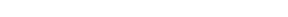Suppose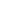is Lipschitz continuous in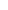and continuous
Continuous function
In mathematics, a continuous function is a function for which, intuitively, "small" changes in the input result in "small" changes in the output. Otherwise, a function is said to be "discontinuous". A continuous function with a continuous inverse function is called "bicontinuous".Continuity of...

in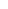. Then, for some value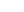, there exists a unique solution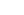to the initial value problem within the range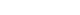.

## Proof sketch

The proof relies on transforming the differential equation, and applying fixed-point theory. By integrating both sides, any function satisfying the differential
equation must also satisfy the integral equation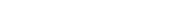A simple proof
Mathematical proof
In mathematics, a proof is a convincing demonstration that some mathematical statement is necessarily true. Proofs are obtained from deductive reasoning, rather than from inductive or empirical arguments. That is, a proof must demonstrate that a statement is true in all cases, without a single...

of existence of the solution is obtained by successive approximations. In this context, the method is known as Picard iteration.

Set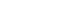and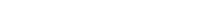It can then be shown, by using the Banach fixed point theorem
Banach fixed point theorem
In mathematics, the Banach fixed-point theorem is an important tool in the theory of metric spaces; it guarantees the existence and uniqueness of fixed points of certain self-maps of metric spaces, and provides a constructive method to find those fixed points...

, that the sequence of "Picard iterates"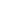is convergent
Limit of a sequence
The limit of a sequence is, intuitively, the unique number or point L such that the terms of the sequence become arbitrarily close to L for "large" values of n...

and that the limit
Limit (mathematics)
In mathematics, the concept of a "limit" is used to describe the value that a function or sequence "approaches" as the input or index approaches some value. The concept of limit allows mathematicians to define a new point from a Cauchy sequence of previously defined points within a complete metric...

is a solution to the problem. Exploiting the fact that the width of the interval where the local solution is defined is entirely determined by the Lipschitz constant of the function,one can assure global existence of the solution, i.e. the solution exists and is unique until it leaves the domain of definition of the ODE.
An application of Grönwall's lemma to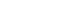where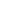and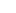are two solutions,
shows that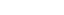, thus proving the global uniqueness (the local uniqueness is a consequence of the uniqueness of the Banach fixed point).

## Detailed proof

Let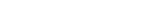be the compact cylinder whereis defined, this is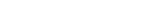and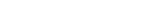.

Let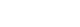,

this is, the maximum slope of the function in modulus. Finally, let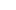be the Lipschitz constant ofwith respect to the second variable.

We will proceed to apply Banach fixed point theorem
Banach fixed point theorem
In mathematics, the Banach fixed-point theorem is an important tool in the theory of metric spaces; it guarantees the existence and uniqueness of fixed points of certain self-maps of metric spaces, and provides a constructive method to find those fixed points...

using the metric on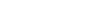induced by the uniform norm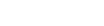We define an operator between two functional spaces of continuous functions, Picard's operator, as follows: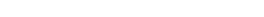defined by: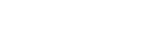.

We impose that it is well-defined, in other words, that its image must be a function taking values on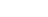, or equivalently, that the norm of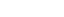is less than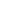,
which can be restated as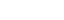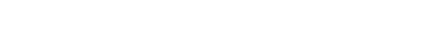The last step is the imposition, so we are impose the requirement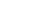Let us impose now the Picard's operator to be contractive under certain hypothesis overthat later on we will be able to omit.

Given two functions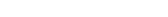, in order to appy the Banach fixed point theorem
Banach fixed point theorem
In mathematics, the Banach fixed-point theorem is an important tool in the theory of metric spaces; it guarantees the existence and uniqueness of fixed points of certain self-maps of metric spaces, and provides a constructive method to find those fixed points...

we want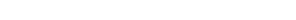So letbe such that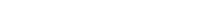then using the definition of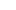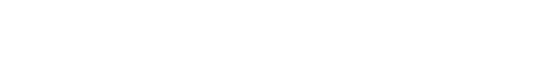Then sinceis Lipschitz with respect to the second variable, we have that: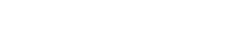This is contractive if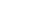.

We have established that the Picard's operator is a contraction on the Banach spaces with the metric induced by the uniform norm. This allows us to apply the Banach fixed point theorem to conclude that the operator has a unique fixed point. In particular, there is a unique function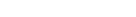such that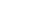This function is the unique solution of the initial value problem, valid on the interval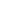wheresatisfies the condition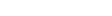.

## Optimization of the solution's interval

Nevertheless, there is a corollary of the Banach fixed point theorem that states that if an operator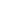is contractive for some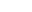then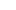has a unique fixed point. We will try to apply this theorem to the Picard's operator. But before doing that, let us recall a lemma that will be very useful to apply the aforementioned corollary.

Lemma: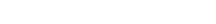We will check this by induction:

For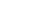we have already seen it, let us suppose it is true for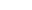and let us check it for: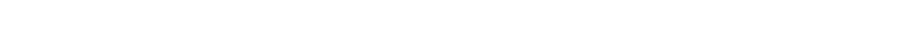.

Therefore, taking into account this inequality we can assure that for somelarge enough, the quantity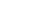and hence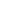will be contractive. So by the previous corollarywill have a unique fixed point. So, finally, we have been able to optimize the interval of the solution by taking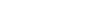.

The importance of this result is that the interval of definition of the solution does eventually not depend on the Lipschitz constant of the field, but essentially depends on the interval of definition of the field and its maximum absolute value of it.

## Other existence theorems

The Picard–Lindelöf theorem shows that the solution exists and that it is unique. The Peano existence theorem
Peano existence theorem
In mathematics, specifically in the study of ordinary differential equations, the Peano existence theorem, Peano theorem or Cauchy-Peano theorem, named after Giuseppe Peano and Augustin Louis Cauchy, is a fundamental theorem which guarantees the existence of solutions to certain initial value...

shows only existence, not uniqueness, but it assumes only that ƒ is continuous in y, instead of Lipschitz continuous. For example, the right-hand side of the equation with initial condition is continuous but not Lipschitz continuous. Indeed, the solution of this equation is not unique; two different solutions are given besides the trivial one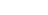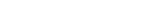Even more general is Carathéodory's existence theorem
Carathéodory's existence theorem
In mathematics, Carathéodory's existence theorem says that an ordinary differential equation has a solution under relatively mild conditions. It is a generalization of Peano's existence theorem...

, which proves existence (in a more general sense) under weaker conditions on ƒ.

## See also

• Frobenius theorem (differential topology)
Frobenius theorem (differential topology)
In mathematics, Frobenius' theorem gives necessary and sufficient conditions for finding a maximal set of independent solutions of an overdetermined system of first-order homogeneous linear partial differential equations...

• Integrability conditions for differential systems
Integrability conditions for differential systems
In mathematics, certain systems of partial differential equations are usefully formulated, from the point of view of their underlying geometric and algebraic structure, in terms of a system of differential forms. The idea is to take advantage of the way a differential form restricts to a...

## External links

The source of this article is wikipedia, the free encyclopedia.  The text of this article is licensed under the GFDL.